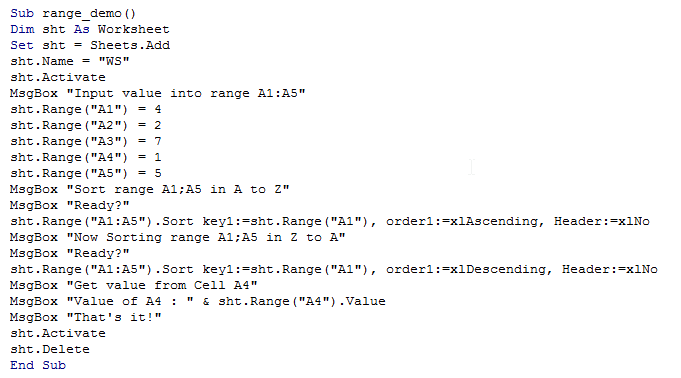Vba range object in Excel

As discussed in previous chapters, a Range is nothing but a collection of Cells within a Sheet.

The Range object is always used along with the Sheets object.

The contents of a sheet can be acessed easily with help of Range object. To do this, it is simply enough to know the address of the cell.

The Range object can be used to either retrive a value from a cell or group of cells, or to write new value in a cell or group of cells.

Simply put, A range represents a cell,row,column or a continous group of cells of any dimension.

The various functionalities and properties of Range object are as discussed below.

Value

The Value property of the range object is used to either get or set value in a cell.

This property is optional as illustrated below in the example

Clear

This method is used to clear a cell or group of cells of its contents.

There are various forms of this method such as ClearContents, Clearformats and so on.

Sort

This property is used to sort a range, usually along a column or row in ascending or descending order.

Formula

This method is used to specify a formula in a cell or group of cells.

The formula expression starts with "=".

Apart from the above mentioned methods and properties there are various other members of range object,

which are very much useful in programming.

The following example illustrates the range object

1. Sub range_demo()
2. Dim sht As Worksheet
4. sht.Name = "WS"
5. sht.Activate
6. MsgBox "Input value into range A1:A5"
7. sht.Range("A1") = 4
8. sht.Range("A2") = 2
9. sht.Range("A3") = 7
10. sht.Range("A4") = 1
11. sht.Range("A5") = 5
12. MsgBox "Sort range A1;A5 in A to Z"
15. MsgBox "Now Sorting range A1;A5 in Z to A"
18. MsgBox "Get value from Cell A4"
19. MsgBox "Value of A4 : " & sht.Range("A4").Value
20. MsgBox "That's it!"
21. sht.Activate
22. sht.Delete
23. End Sub

Editor Screenshot